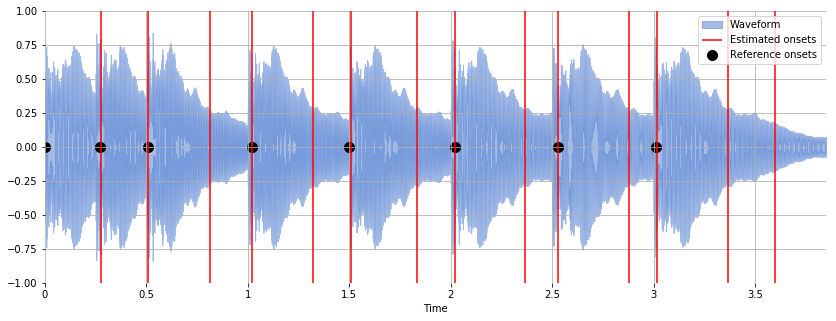# Evaluation Example: Onset Detection¶

Evaluation method: determine which estimated onsets are “correct”, where correctness is defined as being within a small window of a reference onset.

mir_eval finds the largest feasible set of matches using the Hopcroft-Karp algorithm. (See _bipartite_match.)

Let's evaluate an onset detector on the following audio:

In :
y, sr = librosa.load('audio/simple_piano.wav')

Out:

## Detect Onsets¶

Estimate the onsets in the signal using onset_detect:

In :
est_onsets = librosa.onset.onset_detect(y=y, sr=sr, units='time')

In :
est_onsets

Out:
array([0.27863946, 0.510839  , 0.81269841, 1.021678  , 1.32353741,
1.50929705, 1.83437642, 2.02013605, 2.36843537, 2.53097506,
2.87927438, 3.0185941 , 3.36689342, 3.59909297])

In :
ref_onsets = numpy.array([0, 0.270, 0.510, 1.02,
1.50, 2.02, 2.53, 3.01])


Plot the estimated and reference onsets together.## Evaluate¶

In :
mir_eval.onset.evaluate(ref_onsets, est_onsets)

Out:
OrderedDict([('F-measure', 0.6363636363636364),
('Precision', 0.5),
('Recall', 0.875)])

Out of a possible 8 reference onsets, 7 estimated onsets matched, i.e. recall = 7/8 = 0.875.

Out of a possible 14 estimated onsets, 7 reference onsets matched, i.e. precision = 7/14 = 0.5.

The default matching tolerance is 50 milliseconds. To reduce the matching tolerance, adjust the window keyword parameter:

In :
mir_eval.onset.evaluate(ref_onsets, est_onsets, window=0.002)

Out:
OrderedDict([('F-measure', 0.36363636363636365),
('Precision', 0.2857142857142857),
('Recall', 0.5)])